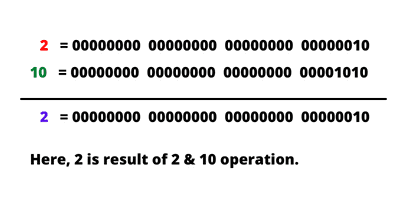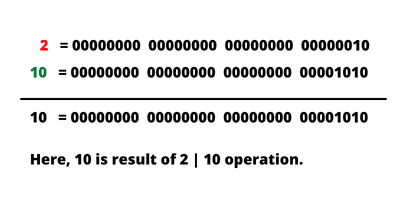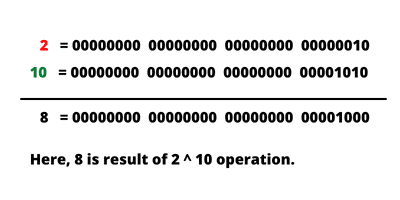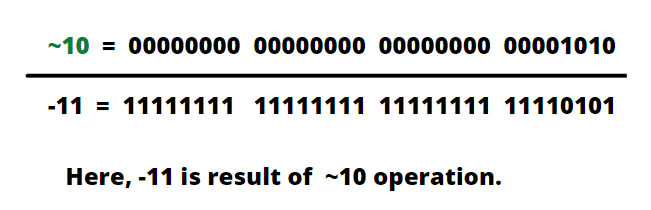# Bitwise Operators in JavaScript | AND, OR, XOR, NOT

JavaScript also supports a set of bitwise operators similar to other programming languages like Java.

Bitwise operators in JavaScript are those operators that works at bit level comprising ones and zeros (i.e., binary representation) rather than decimals or hexadecimals. Here, the term bitwise means to operate on binary numbers.

JavaScript bitwise operators only work with integer numbers that are 32 bits in length.

In bitwise operations, the internal representation of integer numbers is represented by the binary number system. A binary number is represented by two digits, 0 or 1.

Bitwise operators are used in bit-oriented operations. However, these operators are rarely utilized in JavaScript programming because you are dealing with higher-level sequences of bits like numbers or strings.

## Types of Bitwise Operators in JavaScript

JavaScript provides seven bitwise operators to work on individual bits. They are listed in the table below:

OperatorNameType
&Bitwise ANDbinary
|Bitwise ORbinary
^Bitwise XOR (Exclusive OR)binary
~Bitwise NOTunary

Let’s understand all of them one by one in detail.

## Bitwise AND Operator (&)

Bitwise AND operator performs AND operation on each bit of two operands (i.e., numeric values). It compares two bits.

If the corresponding bits of two operands (i.e., both bits are 1) are equal to 1, the result is 1. Otherwise, the resulting bit is 0. The syntax is as follows:

```A & B; // here, A and B are operands.
```

The truth table for this operator is listed in the below table.

ABA & B
000
010
100
111

Consider the following example, where we will AND two numbers (2 and 10).

```var num = 2 & 10; // output is 2.
```

Let’s understand how this works. The below figure shows the 32-bit binary representation of numbers 2 and 10 and the resulting number after performing bitwise AND operation.A truth table is a table that gives the relationship between inputs and output. In AND operation, on multiplying two or more input bits, we get an output bit.

From the truth table, if both compared input bits are 1, we get output 1. Otherwise, we get output 0. From the truth table, On multiplying the individual bits of 2 and 10, we get 2 & 10 = 2.

## Bitwise OR Operator ( | ) in JavaScript

The bitwise OR operator performs a boolean OR operation on each bit of its integer numbers. It is represented by a symbol | called pipe symbol.

In OR operation, each bit of the first operand (number) is compared with the corresponding bit of the second operand. If at least one of the compared bits is 1 then it returns 1. Otherwise, it returns 0. The syntax is as follows:

```A | B
```

The truth table for bitwise OR operator is given below:

ABA | B
000
011
101
111

Consider the truth table of bitwise OR operator. If both compared bits are 0, the output is 0. If anyone bit is 1 in both bits, the output is 1.

Consider the following example, where we will OR two numbers (2 and 10).

```var num = 2 | 10; // output is 10.
```

Let’s understand how this works. The below figure shows the 32-bit binary representation of numbers 2 and 10 and the resulting number after performing bitwise OR operation.On adding input bits of 2 and 10, we get output 10 as shown in the figure.

## Bitwise XOR operator (^)

The bitwise XOR operator performs a boolean exclusive OR (XOR) operation on each bit of its integer numbers. It is represented by a symbol ^ called cap. The general syntax to use XOR operator is as follows:

```A ^ B
```

Bitwise XOR operator compares each bit of the first operand (number) with the corresponding bit of the second operand.

If the odd number of 1’s is in input bits, we get output bit as 1. In other words, if two bits have the same value, the output is 0, otherwise, the output is 1. Look at the below truth table.

ABA ^ B
000
011
101
110

Consider the following example, where we will XOR two numbers (2 and 10).

```var num = 2 ^ 10; // output is 8.
```

Let’s understand how this works. The below figure shows the 32-bit binary representation of numbers 2 and 10 and the resulting number after performing bitwise XOR operation.From the truth table, if we get an odd number of 1’s then the result is 1 otherwise the result is 0. Hence, 2 ^ 10 = 8.

## Bitwise NOT operator (~)

The bitwise NOT operator is basically an inverter. It returns the reverse of its operand or value. It converts all 1s to 0s, and all of the 0s to 1s. Therefore, it is also called unary operator or bit flip operator.

The truth table of bitwise NOT operator is given below:

A~A
01
10

Consider the following example, where we will OR two numbers (2 and 10).

```var num = ~10; // output is -11.
```

Let’s understand how this works. The below figure shows the 32-bit binary representation of numbers 10 and the resulting number -11 after performing bitwise NOT operation.Let’s take a simple JavaScript program, where we will perform all operations based on bitwise operators in JavaScript.

Program code 1:

```<html>
<title>JavaScript Bitwise Operators Example Program</title>
<body>
<script>
let a = 2, b = 10;
document.write("(2 & 10): " +(a & b), "<br>");
document.write("(2 | 10): " +(a | b), "<br>");

document.write("(2 ^ 10): " +(a ^ b), "<br>");
document.write("`10: " +~b);
</script>
</body>
</html>
```
```Output:
(2 & 10): 2
(2 | 10): 10
(2 ^ 10): 8
`10: -11
```

In this tutorial, you learned types of bitwise operators in JavaScript with example programs. Hope that you will have understood all the basic points related to bitwise AND, OR, XOR, and NOT operators.

In the next tutorial, we will discuss string operator in JavaScript with various example programs.